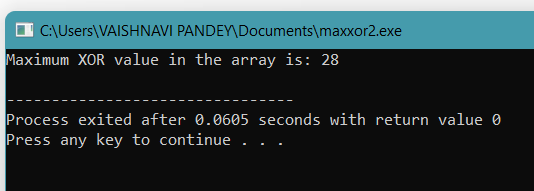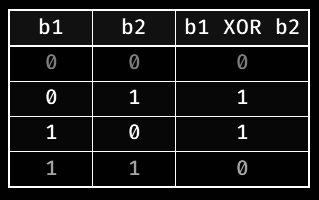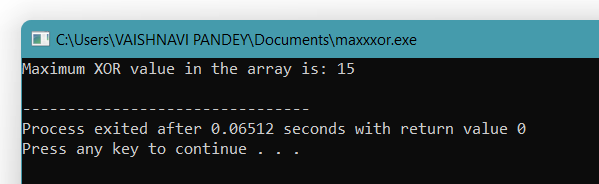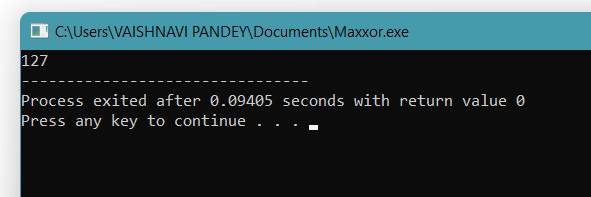# Max XOR of Two Numbers in an Array

## Introduction

We all work hard for our interviews, solve Data structures and algorithms questions, and study CS Fundamentals. Hard work is the key to success!!.

While starting with Data Structures and Algorithms, almost all of us practice questions from Bit Manipulation and Patterns. They are a good starting point to have a grip on programming fundamentals, like loops, control statements, variable scope, functions, etc.  This blog will discuss an important interview question to find the Maximum XOR of two elements in an array. The question, at first sight, seems to be a normal Bit Manipulation problem. But in reality, this problem is an important interview question related to TriesTrie is a sorted tree-based data structure that stores the set of strings. It has the number of pointers equal to the number of characters of the alphabet in each node.

Various approaches to solving this problem are discussed in this blog.

## Problem Statement

The problem on hand is, “Given an integer array of non-negative integers nums, return the maximum result of nums[i] XOR nums[j], where 0 <= i <= j < n..”

Before jumping to the approach straightaway, it is important to analyze the problem statement properly. In an interview, it is recommended to first analyze the problem statement, and if anything doesn't make sense to you in the first go, discuss it with the interviewer. Like in the problem statement above, if the interview doesn't specify non-negative, you must ask if the array has all positives or if it may have some negative elements too, whether the array is sorted or not. Asking such questions gives a positive starting point and forms an impression in front of the interviewer that you are someone who is detail-oriented and focused.

A few important points concluded from the problem statement are:

1. An array of Non-negative integers.
The question clearly rules out the possibility of negative numbers.

2. Finding XOR of element nums[i] and nums[j].
Take a pair of elements from the array and XOR them.

3. The XOR value should be maximum.
Amongst all the XOR values, the maximum value needs to be returned.

Now let us see possible input-output pairs.

Input: arr = {3, 10, 5, 25, 2, 8}

Output:  28

Explanation: Possible pairs are, {3, 10}, {3, 5}, {3, 25}, {3, 2}, {3, 8}, {10, 5}, {10, 25}, {10, 2}, {10, 8}, {5, 25},{5,2}, {5, 8}, {25, 2}, {25, 8}, {2, 8}.

5 XOR 25 will give the maximum result, i.e., 28.

Input: arr = {8, 10, 2}

Output: 10

Explanation: Possible pairs are, {8, 10}, {8, 2}, {10, 2}. 8 XOR 2 will give the maximum result i.e. 10.

You may solve a slight variation of this problem on your own before moving on to the solution.

## Approach 1: Brute Force Approach

In an interview, always start with the brute force approach, the one that is not optimized at all. It’s the immediate approach that comes to our minds while reading the problem statement.

The brute force approach for this question is to generate all the pairs from the array and then find the maximum xor value.

### Algorithm

1. Initialize a variable maximumXOR value with 0.
2. Generate all the possible pairs of the given array. Computer XOR value of each of the pairs, currentXOR. At each iteration, compare the currentXOR and maximumXOR. The maximumXOR will hold the maximum of all the XOR values.
3. Return the value of the maximumXOR.

### Java Implementation

The implementation of the above approach in Java is:

``````// Brute Force Approach for finding the maximum XOR
// value from all the pairs in an array
public class Solution{

// Method to find the maximum XOR value
static int maxXOR(int[] arr, int size)
{
int maximumXOR = 0;

// Iterate over all the pairs of an array
for(int i = 0; i < size; i++)
{
// This will hold the value of the XOR of the element arr[i] and arr[j]
int currentXOR = 0;
for(int j = i + 1; j < size; j++)
{
currentXOR = arr[i] ^ arr[j];
maximumXOR = Math.max(maximumXOR, currentXOR);
}
}

return maximumXOR;
}
public static void main(String[] args) {
int[] arr = {3,10,5,25,2,8};
System.out.println("Maximum XOR value from arr is: ");
System.out.println(maxXOR(arr, arr.length));

int[] arr2 = {14, 70, 53, 83, 49, 91, 36, 80, 92, 51, 66, 70};
System.out.println("Maximum XOR value from arr2 is: ");
System.out.println(maxXOR(arr2, arr2.length));

}
}``````

Output

``````Maximum XOR value from arr is:
28
Maximum XOR value from arr2 is:
127``````

### C++ Implementation

``````#include <bits/stdc++.h>
using namespace std;

int max_xor(int arr[], int n)
{

int maxXor = 0;

// Calculating xor of each pair
for (int i = 0; i < n; i++) {
for (int j = i + 1; j < n; j++) {
maxXor = max(maxXor,
arr[i] ^ arr[j]);
}
}
return maxXor;
}
int main()
{

int arr[] = {3,10,5,25,2,8};
int n = sizeof(arr) / sizeof(arr);

cout << "Maximum XOR value in the array is: "<<max_xor(arr, n) << endl;
return 0;
}``````

Output### Python Implementation

``````from typing import List

def findMaximumXOR(self, nums: List[int]) -> int:
result = 0
for i in range(0, len(arr)):
for j in range(1, len(arr)):
calc = arr[i] ^ arr[j]
result = max(result, calc)

return result

arr = [14, 70, 53, 33, 49, 91, 36, 80, 92, 51, 66, 70]
print(findMaximumXOR(arr, arr))``````

### Complexity Analysis

Time Complexity: The time complexity of the above program is O(N^2).

Space Complexity: The space complexity of the above program is O(1).

The above approach with O(N^2) complexity is not a good choice for solving this problem. In an interview, when such a situation arises that you can’t figure out what to do now, start examining the input-output pairs, and in questions like these where a particular operation is to be performed, examine the result of the binary operation to be performed.

Proceeding in this way, The truth table of XOR is:It can be deduced from the above table that, We can maximize the bitwise ‘xor’ value for any two integers by taking their bits at ‘i- th’ position as 1 and 0(Case 3) or 0 and 1(Case 2).

For an integer 'X' in binary representation, we start from its most significant bit and try to find a corresponding number 'Y' from available numbers such that bits at the current position of 'X' and 'Y' are different, in this way we could maximize the bitwise xor value for 'X.'

For example, consider an array of 5 numbers, {3, 10, 5, 25, 2}. The binary representation of each of the numbers is, {00011, 01010, 00101, 11001, 00010}; for the sake of simplicity, I have taken only 5 bits in binary representation. Now let’s construct the maximum XOR starting from the leftmost bit; the absolute maximum by taking 5 bits is 11111.

• The maximum XOR value could be of the format (1****). In the above example, 25 or 11001 is of the format (1****). Now pair 25 with another number starting from 0 leftmost bit, such that the maximum XOR value becomes (1****).
• In the next step, find the pair of numbers such that the maximum XOR value is of the format (11***). For this, consider all the prefixes of length 2 and check if there is a pair such that XOR of them equals 11.
• 3 = (00***)
• 10 = (01***)
• 5 = (00***)
• 25 = (11***)
• 2 = (00***)

It can be easily deduced that we can pair 3 and 25, 2 and 25, and 5 and 25.

• In the next step, find the pair of numbers such that the maximum XOR value is of the format (111**). For this, consider all the prefixes of length 2 and check if there is a pair such that XOR of them equals 111.
• 3 = 000**
• 10 = 010**
• 5 = 001**
• 25 = 110**
• 2 = 000**

It can be easily deduced that both 3 and 2 have all the three bits set as 0, but there is no number with all the three bits set as 1. The maximum XOR value possible is thus of the format, (11***).

Repeat the same process for all the bits of a number.

So for a 32-bit integer, we just need to iterate through all its bits and check for a corresponding integer from another array such that their xor value is maximum.

### Algorithm

1. Calculate the number of bits, maxBits to be used. It is the length of the maximum number in the binary representation.
2. Loop from i = maxBits - 1 to i = 0, i.e. from the leftmost bit to the rightmost bit. In each iteration, do the following
1. Left shift maxXOR to free the next bit.
2. The variable currXOR is set to maxXOR | 1 by setting 1 in the rightmost bit of maxXOR. Note that | is the bitwise inclusive OR operator.
3. Compute all the possible prefixes of length maxBits - i by iterating over arr.
1. Put in the HashSet, the prefix of the current number of length maxBits - 1, this is done using arr >> i in the code.
4. Now iterate through the prefix, and check if currXOR could be achieved using p1^p2.
1. By Using the self-inverse property of XOR, currXOR = p1^p2 can be rewritten as p1 == currXOR^p2.
So simply check for each p, if currXOR^p is in the prefix HashSet or not. If it is there, then maxXOR will be equal to the currXOR.
5. Return maxXOR.

### Java Implementation

The implementation of the above approach is given below:

``````import java.util.HashSet;
import java.util.Set;
class MaximumXOR{

public static int findMaximum(int[] arr){

// The first step is to find the maximum number in
// the array. Because we will take the number
// of bits which are there in the binary
// representation of the maximum number

// Finding the maximum number of the array
int maxNum = arr;
for(int i = 0; i < arr.length; i++){
maxNum = Math.max(maxNum, arr[i]);
}

// Finding the number of bits in the maximum
// number
int maxBits = (Integer.toBinaryString(maxNum)).length();

int maxXOR = 0;
int currXOR;

Set<Integer> prefix = new HashSet<>();

// Step 2: Iterate over the bits of the number
for(int i = maxBits - 1; i > -1; --i){

// Left Shift maxXOR by 1 bit so that the next bit
// can be reached
maxXOR <<= 1;

// Set 1 in the smallest bit
currXOR = maxXOR | 1;

prefix.clear();

// compute all possible prefixes of length maxBits - 1
// in binary representation
for(int num : arr){
}

// Update the maxXOR, if any two of the prefixes
// could result in currXOR

for(int j: prefix){
if(prefix.contains(currXOR^j)){
maxXOR = currXOR;
break;
}
}
}
return maxXOR;
}
public static void main(String[]args){
int[] nums = {3, 10, 5, 15, 2, 8};
System.out.println("Maximum XOR value in the array, arr is");
System.out.println(findMaximum(nums));
}
}``````

Output

``````Maximum XOR value in the array, arr is
15``````

### C++ Implementation

``````#include <bits/stdc++.h>
using namespace std;

int FindMaxXor(int arr[], int n)
{
int maxx = 0, mask = 0;

set<int> s;

for (int i = 30; i >= 0; i--)
{

// setting the i'th bit in mask

for (int i = 0; i < n; ++i) {

}

int newMaxx = maxx | (1 << i);

for (int prefix:s) {

if (s.count(newMaxx ^ prefix)) {
maxx = newMaxx;
break;
}
}
s.clear();
}

return maxx;
}

// Driver Code
int main()
{

int arr[] = {3, 10, 5, 15, 2, 8};
int n = sizeof(arr) / sizeof(arr);

cout << "Maximum XOR value in the array is: "<<FindMaxXor(arr, n) << endl;

return 0;
}``````

Output### Python Implementation

``````from typing import List
"""
let ni and nj be the numbers such that xor between them produces maximum
value among other pairs. let m be the max value.

m = ni ^ nj
=> m ^ nj = (ni ^ nj) ^ nj
=>        = ni ^ (nj ^ nj) # xor is associative
=>        = ni ^ 0
=>        = ni

so, m ^ nj = ni

We note that m is 31-bit integer so we guess bits of m and and with each nj,
we check if the combination of m and nj will produce ni.

So time complexity becomes O(32N) = O(N)
:param nums:
:return:
"""

def findMaximumXOR(self, nums: List[int]) -> int:
for i in range(32)[::-1]:
prefixes = {n & mask for n in nums}

tmp = m | (1 << i)

if any(prefix ^ tmp in prefixes for prefix in prefixes):
m = tmp
return m

arr = [3, 10, 5, 25, 2, 8]
print(findMaximumXOR(arr, arr))``````

### Complexity Analysis

The time complexity of the above program is O(N*log(M)), where N is the size of the array and M is the maximum element of the array.

The space complexity is O(N).

Before moving on to the next approach, it is necessary to understand the Trie Data Structure and the implementation of various operations on Trie. You must refer to the blog for a better understanding.

## Approach 3: Using Tries

As a next step, the interviewer will ask you to optimize the above solution in terms of time and space complexity or may directly ask you to solve it using Tries. In fact, the majority of the interviewers ask this question to check the candidate's thinking ability and to test the knowledge of Tries(See Using Trie in Data Structure). The intuition for this approach is the same as the above one, i.e., we can maximize the 'XOR' value of any two integers by taking their bits at the ith position as 1 and 0 or as 0 and 1,

The idea is to insert the binary representation of the numbers in the array arr[] into a Trie. Iterate through the binary representation of all the numbers in the trie and if the current bit is 0, then find the path with value 1 and vice-versa. Keep updating the maximum value for each number in the process.

### Algorithm

1. Initialize the maximumXOR as 0.

2. Create a trie data structure to store the binary representation of 32-bit integers of the array. While insertion, if the current bit is 0, then create a node in the left. Else create a node in the right of the current head node.

3. Linearly traverse the given array, and for each element of the array.Initialize currentXOR as 0.
Traverse the binary representation of the element stored in trie.

If the ith bit is 1 and node->left exists then update the value of currentXOR as currentXOR + pow(2, i) and update the node as node->left.

Otherwise, update node = node -> right.If the ith bit is 0 and node->right exists then update the value of currentXOR as currentXOR + pow(2, i) and update the node as node->right. Otherwise, update the node and node->left.

4. For each array element, update the maximumXOR if the maximumXOR is greater than the currentXOR.

5. Print the value of the maximumXOR.

### Java Implementation

The implementation of the above approach is:

``````class XorUsingTrie {
static class Node {
Node left;
Node right;
};

// A utility method to insert the 32 bit binary representation of a number
// x in the trie.
static void insertTrie(int x, Node head) {

for (int i = 30; i >= 0; i--) {
// Finding the bit at the ith position
int val = (x >> i) & 1;

// if the current bit is 0, it will be stored to
// the left of curr node.
if (val == 0) {

// if the curr.left is null
if (curr.left == null) {
curr.left = new Node();
}

curr = curr.left;
}

// if the bit at the ith position is 1, it will
// be stored to the right of the curr node.
else {
// If the curr.right is null
if (curr.right == null) {
curr.right = new Node();
}

curr = curr.right;
}
}
}

// Function to find the maximum XOR value amongst
// all the pairs formed in an array, arr[].
static int findMaximum(int[] arr, int size) {

// Head node of the trie data structure.

// Step 1: Insert each element to the trie
for (int i = 0; i < size; i++) {
}

// Step 2: A variable to store the maximum XOR value
int maximumXOR = 0;

// Step 3: Traverse the given array, find the ith bit
// and update the value of the currentXOR.

for (int i = 0; i < size; i++) {
int currentXOR = 0;
// Note that M is 1073741824
// The decimal representation of M is
// 1000000000000000000000000000000
int M = (int)Math.pow(2, 30);

for (int j = 30; j >= 0; j--) {

// Finding the ith bit
int val = (arr[i] >> j) & 1;

// if the ith bit is 0
if (val == 0) {
if (curr.right != null) {
currentXOR = currentXOR + M;
curr = curr.right;
}

else {
curr = curr.left;
}
}

// if the ith bit is 1
else{
if(curr.left != null){
currentXOR += M;
curr = curr.left;
}

else{
curr = curr.right;
}
}

// Update the value of M to M/2 to find
// the next set bit
M = M/2;
}

maximumXOR = Math.max(maximumXOR, currentXOR);
}
return maximumXOR;
}

public static void main(String[] args) {
int[] nums = {3, 10, 5, 15, 2, 8};
System.out.println("Maximum XOR value from nums is: ");
System.out.println(findMaximum(nums, nums.length));

int[] arr = {14, 70, 53, 33, 49, 91, 36, 80, 92, 51, 66, 70};
System.out.println("Maximum XOR value from arr is: ");
System.out.println(findMaximum(arr, arr.length));

}
}``````

Output

``````Maximum XOR value from nums is:
15
Maximum XOR value from arr is:
127``````

### C++ Implementation

``````#include <bits/stdc++.h>
using namespace std;

class Node {
public:
Node* left; // 0
Node* right; // 1

};

class Solution {
public:
Node* root;

Solution() {
root = new Node();
}

void insert(int n) {

Node* temp = root;

for (int i = 31; i >= 0; i--) {
int currentBit = (n >> i) & 1;

if (!currentBit) {
if (!temp->left) temp->left = new Node();
temp = temp->left;
}

else {
if (!temp->right) temp->right = new Node();
temp = temp->right;
}

}
}

// O(1) loop
int helper(int val) {
int ans = 0;
Node* temp = root;

// 0 -> move right; else vice versa
for (int i = 31; i >= 0; i--) {
int currentBit = (val >> i) & 1;

if (!currentBit) {
if (temp->right) {
temp = temp->right;
ans += (1 << i);
}
else temp = temp->left;

}
else {
if (temp->left) {
temp = temp->left;
ans += (1 << i);
}
else temp = temp->right;

}

}

return ans;

}

// O(n)
int findMaximumXOR(vector<int> &arr) {
int n = arr.size();
int res = 0;
for (int i = 0; i < n; i++) {

int value = arr[i];
insert(value);

int curAns = helper(value);

res = max(curAns, res);
}

return res;
}
};

int main()
{
int arr[] = {14, 70, 53, 33, 49, 91, 36, 80, 92, 51, 66, 70};
int n = sizeof(arr) / sizeof(arr);
Solution s;

vector<int> v(arr, arr + n);
cout << s.findMaximumXOR(v);

return 0;
}``````

Output### Python Implementation

``````from typing import List
from collections import defaultdict

class TrieNode:
def __init__(self):
self.children = defaultdict(TrieNode)
self.val = 0

class Trie:
def __init__(self):
self.root = TrieNode()

def insert(self, binary):
cur = self.root
for char in binary:
cur = cur.children[int(char)]

def search(self, binary) -> int:
result = 0
cur = self.root
for char in binary:
opposite = 1 - int(char)
if opposite in cur.children:
result = (result << 1) | 1
cur = cur.children[opposite]
else:
result = (result << 1)
cur = cur.children[int(char)]
return result

def findMaximumXOR(self, nums: List[int]) -> int:
trie = Trie()
result = float('-inf')
# format binary string in length of  max num
maxLen = len(bin(max(nums))[2:])
for num in nums:
key = bin(num)[2:].zfill(maxLen)
trie.insert(key)
result = max(result, trie.search(key))
return result

arr = [14, 70, 53, 33, 49, 91, 36, 80, 92, 51, 66, 70]
print(findMaximumXOR(arr, arr))``````

### Complexity Analysis

The time complexity of the above approach is O(32*N) or precisely O(N), where N is the size of the array.

The space complexity of the above approach is O(32*N).

Let's take a look at a quick video of the implementation of the program with the proper concept to understand it better.

### How to find the maximum XOR pair value in an array?

The maximum XOR value in an array can be found by the following methods.

• Brute Force Approach
• Using Tries

### What is the XOR of two numbers?

The XOR logical operation, or exclusive or, takes two boolean operands and returns true if and only if the operands are different. Thus, it returns false if the two operands have the same value.

The truth table of XOR is:### When XOR value is maximum?

For a given number N, the maximum possible XOR value is 2^log2(N) + 1. That is the value when all bits of N are turned to 1.

## Conclusion

This blog discussed an important interview question and its implementation using various approaches.

#### Recommended Problems

If you are preparing for your interview and are looking for a complete guide for interview preparation, you must check out Guided Paths on Codestudio curated by experts placed in Big Tech Giants. Remember, Practice is the Key !!

Happy Coding Ninja!!